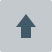# Team:TJUSLS China/Modeling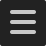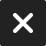Modeling### Part1 Reaction kinetics

There are three reasons we choose two steps, adsorption and reaction, to describe the process of PET enzymatic hydrolysis reaction:
First, the adsorption process has a greater impact on the PET enzymatic hydrolysis process which we can’t ignore.
Second, the combination of the two parts can solve the problem that the Michaelis-Menten equation is not suitable for heterogeneous reactions.
Third, the effect of reaction area in the hydrolysis process can be well considered by taking the adsorption process into account.
In this part, we choose the Langmuir isothermal adsorption equation to describe the first step in the reaction process, the combination of the PET film and the PET enzyme. The general adsorption image of this equation in a typical solution is shown in picture 1: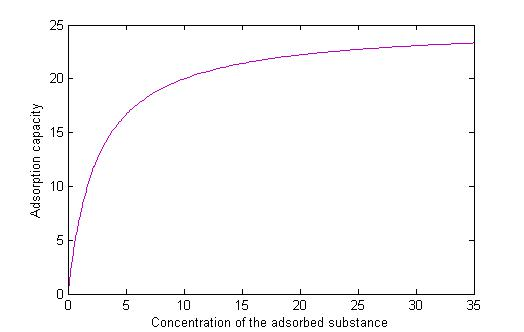Picture 1: The image of the Langmuir adsorption.

Here we assume that the PET enzyme adsorption process in accordance with the adsorption process in the picture above. This isothermal adsorption equation is suitable for the case where there is only one adsorbed species, while the PET adsorption process is just this one. In order to apply this equation to solve the reaction kinetics problem well, some assumptions are given follows:
A.The solid surface of PET is smooth.
B.The adsorption of PET enzyme on the PET-solid-surface is monomolecular layer.
C.No intermolecular forces between the PET enzyme molecules.
D.The adsorption process of PET enzyme on the PET-solid-surface will come a dynamic equilibrium after a certain time.
According to the Langmuir theory, the adsorption isotherm equation is: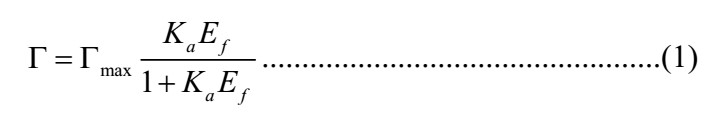In the above equation, Γ is the quality of PET enzyme adsorption by unit quality PET, g; Γmax is the maximum adsorption of PET enzyme by unit quality PET, g; Ka is the adsorption dissociation constant, mL/g; Ef is the concentration of free PET enzyme in the solution, g/mL.
In general, we use the double reciprocal method to find the adsorption constant of the Langmuir equation. It’s used in this situation as well, so the double reciprocal equation is: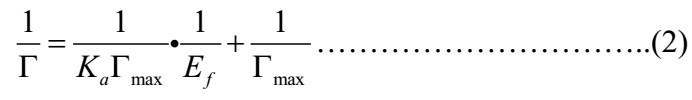So if we have the experimental data by experimenting with quartz crystal microbalance to detect the adsorption capacity of PET, we can get the constant Γmax and Ka through the equation above. While it’s no time for us to do so many adsorption process experiments, so it’s the other way we need to seek in order to solve the reaction kinetics problem well.
We seek the ultimate goal first now, and it’s well known that we want to get the reaction kinetics equation. So the valve of the constant Γmax and Ka is not wanted if we can get the reaction kinetics equation finally through another way. First of this, let us see the association of the constant Γmax and the reactant concentration it will be used later. The dimensionless adsorption capacity is defined as the following formula: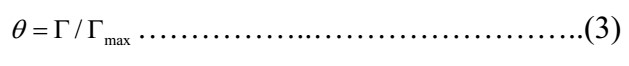So, in the above equation, θ is represents the dimensionless adsorption capacity. So the Langmuir adsorption equation can be converted into the next form: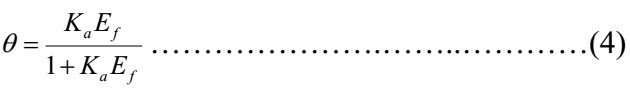The maximum adsorption capacity of the solution will be avoided by using this model. And as we always know, the maximum adsorption capacity is certainly proportional to the area of the PET film, so the concentration of the combination state of the PET enzyme and PET film in the whole system is related to the PET film area and dimensionless adsorption capacity. Here, we use the character PET•S represent the middle state of PET enzyme adsorption on the surface of PET sheet. So the concentration of this PET•S can be achieved through the following equation: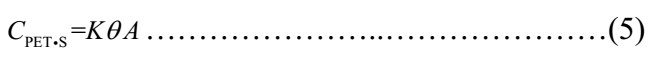While in the above equation, K is a constant connecting the three characters and A represents the area of the PET film. In this way, we put the Langmuir adsorption equation linked with the second step of hydrolysis reaction process, here, the equation above is the key to connect the two step, and the valve of the CPET•S will be used in the next part. It avoid the solving process of the Γmax needing the adsorption experiment by quartz crystal microbalance which would cost a lot of time.
1.2 PET enzymatic hydrolysis process
1.2.1 Abstract
The model of PET enzymatic hydrolysis process is made up of two parts. It’s known that the general enzymatic reaction rate is far faster than diffusion rate, so, in this process, the hydrolysis reaction is very important as well as the diffusion adsorption process.
According to combining the Langmuir equation and the reaction rate equation we get a heterogeneous kinetic model. In this model, only the PET enzyme binds to the PET sheet and the PET is insoluble in the solution. While, in fact, the product of the hydrolysis reaction, BHET and MHET shown a competitive inhibition of the hydrolytic activity of PET enzyme against PET sheet, which would decrease the free concentration of free enzyme in the solution according to Markus Barth. So the kinetic equation eventually needs to be corrected which we will cover in the next section.
1.2.2 Model
In this part, our goal is to deduce the chemical reaction rate. We make some reasonable assumptions in order to simplify the derivation process because of the complex enzymatic reaction process. Here are the hypothesizes:
A. The change of the area of the PET sheet is assumed to be negligible due to the slow rate of PET decomposition.
B. The reverse reaction rate is assumed to be zero as the quality of cis-reaction products is negligible compared to the amount of solution due to the slow cis-reaction rate.
C. The changes in the quality of water can be excluded because of the large excess of aqueous solution.
D. The time to achieve the equilibrium of the adsorption reaction can be ignored. Because the reaction time relative to the adsorption time is so long that the time to achieve the adsorption equilibrium can be negligible, while, after reaching the maximum adsorption quantity, new PET enzyme will be adsorbed on the PET sheet soon whenever there is some PET enzyme reaction with PET to release the products.
E. The enzymatic reaction is carried out only on the solid surface of the PET sheet, and the PET enzyme won’t enter the interior of the PET, and the solubility of the PET in the solution is zero.
F. The concentration of PET enzyme in solution is assumed to be constant.
There are three main steps in the whole process on the basis of the given assumptions above, the first step is the former part which describes the adsorption process and the second step is the effective combination for enzyme catalytic reaction of the PET film and the PET enzyme on the basis of the physical adsorption. At last, the third step is the hydrolysis reaction and the three-step reaction equations as follows: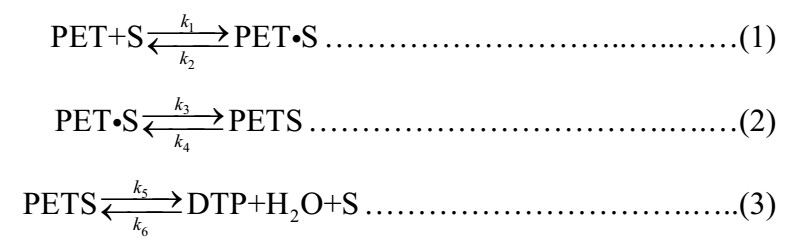In the above equation, the meaning of each symbol represents as follows:
S: PET enzyme
PET•S: The middle state of PET enzyme adsorption on the surface of PET sheet.
PETS: The binding state of PET in the PET enzyme active site.
DTP: The main product of PET hydrolysis, dimethyl terephthalate.
k1-6: The reaction constant of each reaction equation.
Here is the explanation of the symbols involved in the later works in order to know the derivation well. As we all know, the symbol C means the concentration of the material, so we can get that CPET•S is the concentration of the adsorption combination state of the PET film and the PET enzyme; CPETS is the concentration of the effective combined of the PET film and the PET enzyme; CS is the concentration of the free PET enzyme in the solution and CDTP is the concentration of the product, dimethyl terephthalate. We needn’t to take the reaction rate of the first reaction equation into account because it has been calculated in the adsorption part and the differential equation of the remaining two reaction rate as follows: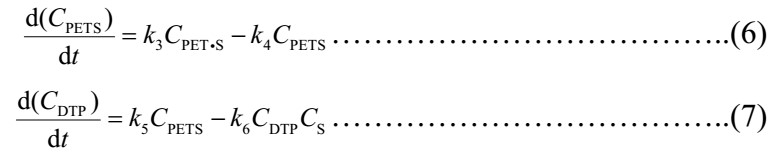so the following equation can be gotten by putting the relationship equation of the concentration of PET•S and the PET film’s area into the above differential equation: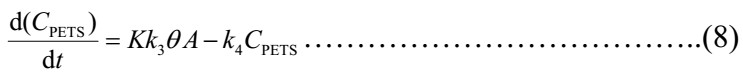And combine the above equation and the equation of dimensionless Langmuir adsorption equation, we can get: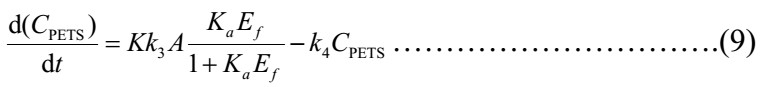Now, let’s analyze the parameters of the above equation. It’s obvious that K, k3, Ka are constant in the system, while, in a fixed experiment, the area of the PET sheet and the concentration of the PET enzyme are unchangeable according to hypothesizes above, so the right part of the equation above is a constant, B. So we can get: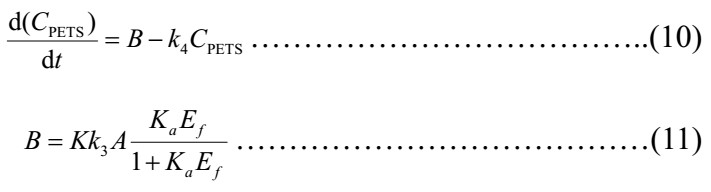According to our hypothesis, when the PET•S is decompose into the reaction products there will soon be a new PET binding on the PET film, so in the whole reaction process, the amount of the PETS won’t change at all, and we can get the differential equation being zero.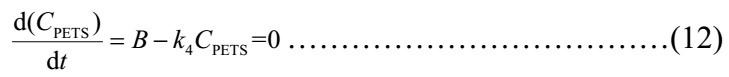So the equation of the concentration of PETS is: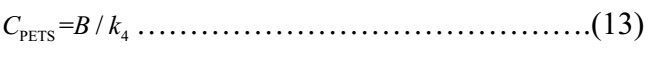While putting it into the equation-7 we can get: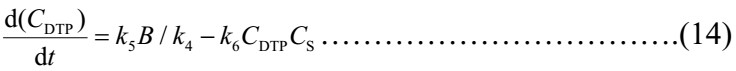and because of the assumptions as the reverse reaction rate is zero, so the constant valve of k6 is zero and the equation-14 can change into: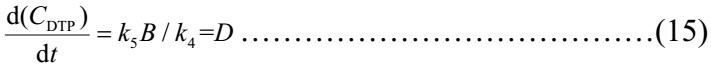The above differential equation is the final rate equation of the whole reaction process, and from the equation-15 we can see that the reaction rate is a constant, D. And the reaction rate constants were different at different PET concentrations and different PET films.
1.2.3 Model modification
As can be seen from the above reaction kinetics model, the whole reaction rate is a constant after reaching the adsorption equilibrium. But the reaction rate shouldn’t be a constant according to our experimental results in the whole process which indicates that the reaction products has a certain effect on the process. According to Markus Barth, we can know that the products such as: TPA, MHET, EG and BHET have a competence of combining with the PET enzyme, what’s more, MHET and BHET are powerful in this. The illustration is shown below by picture-2: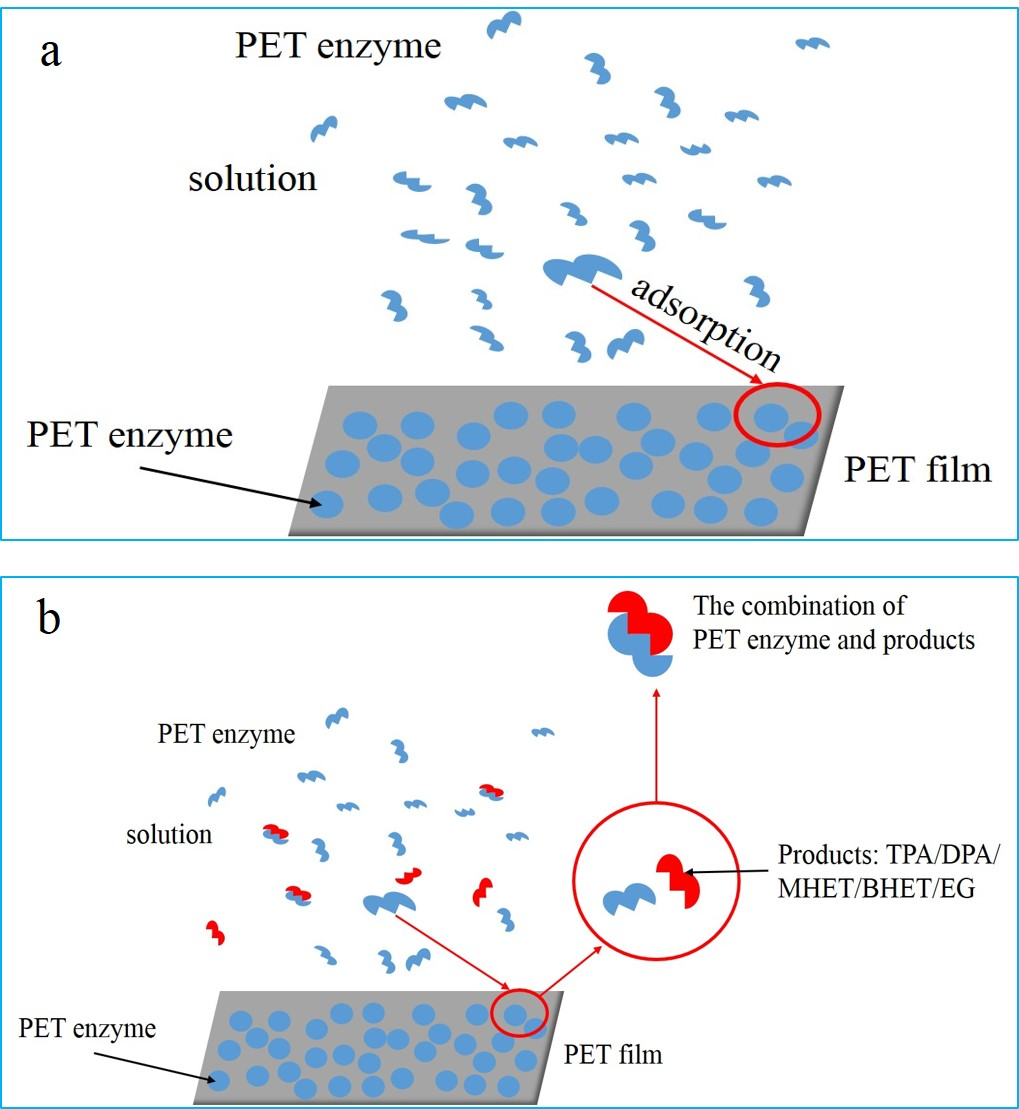Picture 2:a.The state of the adsorption without hydrolysis reaction happened. b. Schematic diagram of the combination of the PET enzyme and reaction products while the hydrolysis reaction is happening.

When the enzyme adsorbed on the PET film is released after the reaction, a new PET enzyme will be adsorbed on the PET immediately from the picture 2, so the adsorption equilibrium is not broken. While the combination of the PET enzyme and the products reduces the PET enzyme concentration in solution which moves the adsorption equilibrium to deduce the maximum adsorption capacity and equilibrium adsorption capacity. So the valve of the character, B, is not a constant because the valve of the concentration of PET enzyme, Ef, is changeable with the reaction time. Here we only consider the main obstacles, BHET and MHET, even though we ignore these by-products because they are so few, but their influence on the adsorption can’t be excluded. We take the character I as the damping factor of all products. So we can get: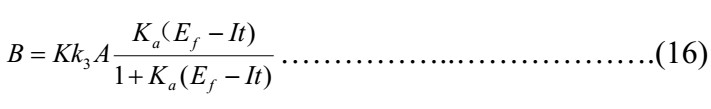So we can get the modified reaction rate equation: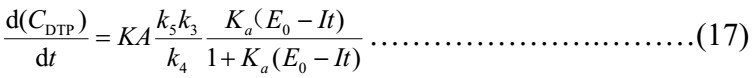We make: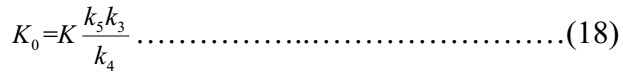So the final reaction rate equation is: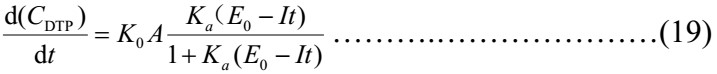The parameter valves can be given according to the literature and experimental data:

Table 1: The valve table of the each parameters.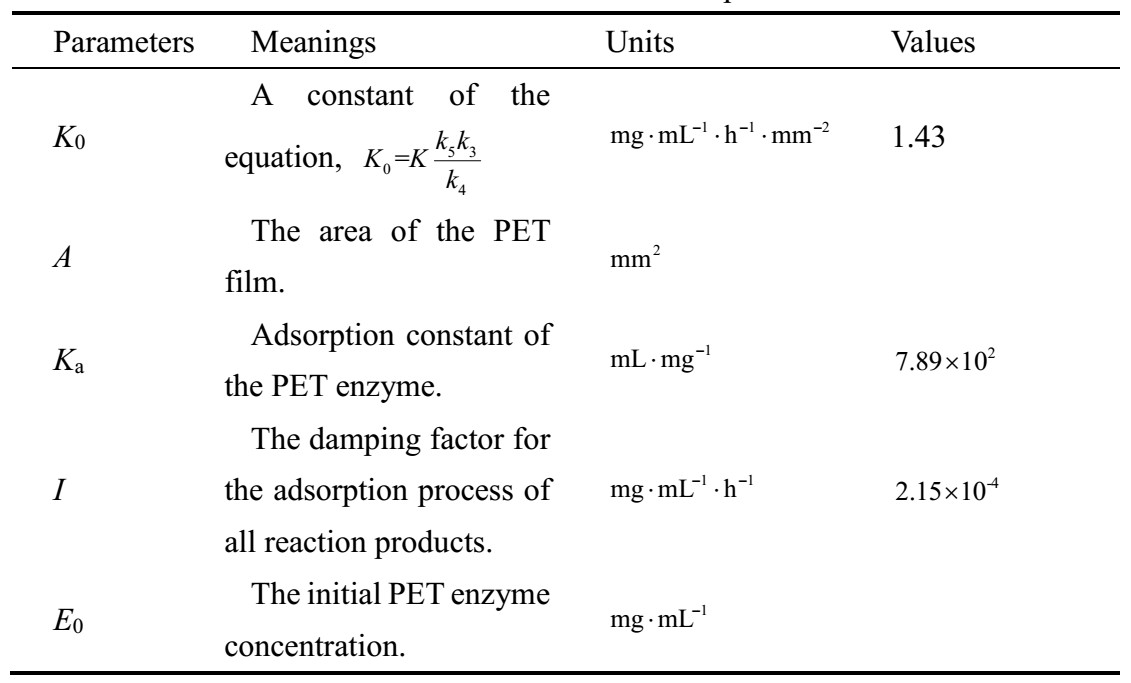So the image of the reaction rate with the time under the condition of the A=28.27mm2, and E0=4×10-3 is shown in the picture 3.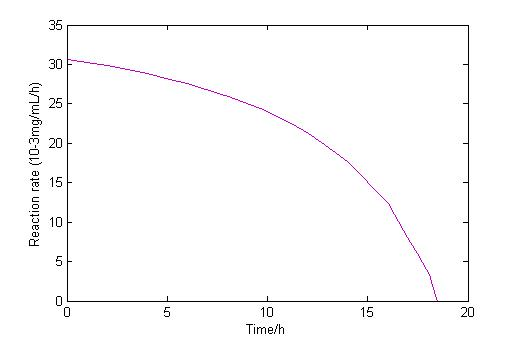Picture 3: The image of the reaction rate with the time.

From the picture above, we can get that the reaction rate will decrease with the time because of the reaction products’ prohibition. We can get that when it’s 18 hours later, the reaction rate is zero which is in accordance with the experimental results that when we extend the reaction time the products won’t increase. And through this reaction rate equation we can get different reaction rate in the condition of different PET film and PET enzyme initial PET enzyme concentration.

### Part 2: The optimum condition of experiments

2.1 Introduction
In our case, in order to predict the optimum condition of recombination cell decomposing PET, it was carried out the multivariate nonlinear regression analysis model. The actual experimental data was analyzed by using the nlinfit function in MATLAB’s Statistics Toolbox. The prediction results showed in good agreement with actual value, and the model is well suitable for the actual system.
2.2 Design and prediction set
Multivariate nonlinear regression is a form of regression analysis in which observational data are modeled by a function which is a nonlinear combination of the model parameters and depends on multiple independent variables.
We set three variables as the reaction conditions in our model, which are the time(x1) and temperature(x2) of protein-induced expression and the cell concentration(x3). We consider that the reaction efficiency correlates with the protein-induced expression time logarithmically, and correlates with the temperature of protein-induced reaction and cell concentration quadratically. So the fitting equation is designed as follows.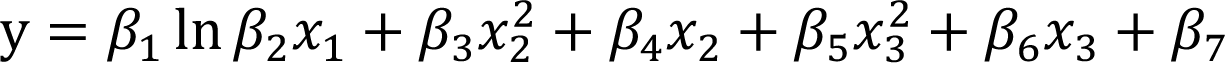In this case, the statistical parameters were obtained by this regression-based approach. The considered tolerance parameter for minimum acceptable tolerance was the default value of 0.01. The forward stepwise procedure was the strategy for variable selection.
The experimental data of one experiment group we used is shown in table2.

Table 2: Relative Enzyme Activity of E-coli with INP(ice nucleation protein)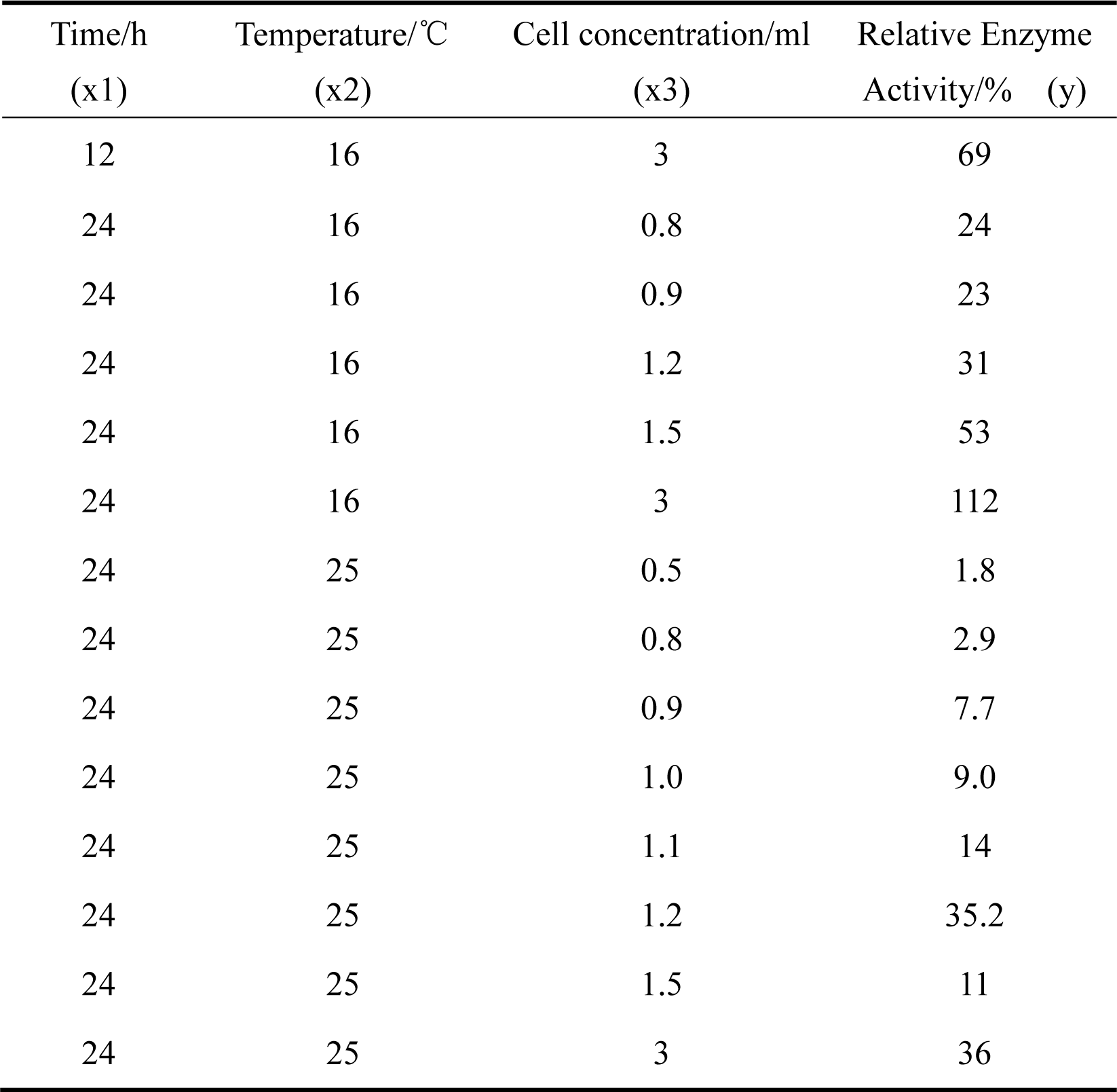2.3 Results and analysis
The results of our model are as follows. And this model only can be used to predict the optimum condition of E-coli cell which is surface displayed by INP (ice nucleation protein).
These are the calculated coefficient in our fitting equation:So our fitting equation has the following form:
y=27.25 ln⁡〖5.23x_1 〗+0.16x_2^2-9.82x_2+1.73x_3^2+18.81x_3-0.81
with the residual error is [0, -8.02, -11.19, -9.92, 5.03, 24.11, 4.88, 3.36, 0.27, 3.03, 21.95, -9.29, -24.21].
And the predicted y is [69.0, 32.0, 34.2, 40.9, 47.9, 87.9, 2.0, 4.3, 8.7, 10.9, 13.2, 20.3, 60.2], with the confidence intervals is [37.31, 20.84, 18.90, 18.57, 21.85, 28.59, 28.31, 16.78, 14.95, 15.69, 17.05, 21.83, 28.51] in the confidence probability of 95%.
We made a 4-dimension picture to show the relationship between the selected three conditions and the PET decomposition efficiency using our fitting model. The red color means the high decomposition efficiency, meanwhile blue means the low decomposition efficiency. And the x-label means the temperature of protein-induced (from 16℃ to 25℃), y-label means the time of protein-induced (from 12h to 24h), z-label means the cell concentration (from 0.5mL to 3mL). Figure1 is a surf picture of our model, while Figure2 is a slice picture for our convenience in observing the details.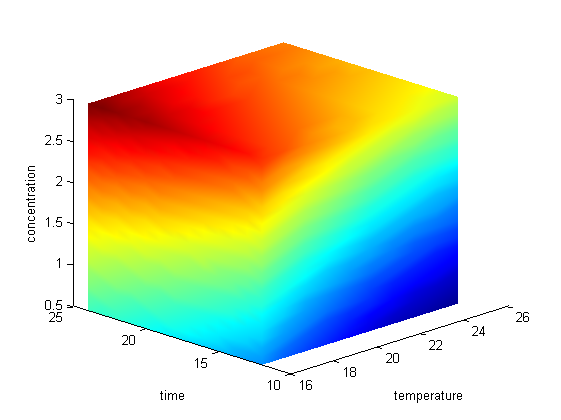Figure4: 4-D surf picture of Relative Enzyme Activity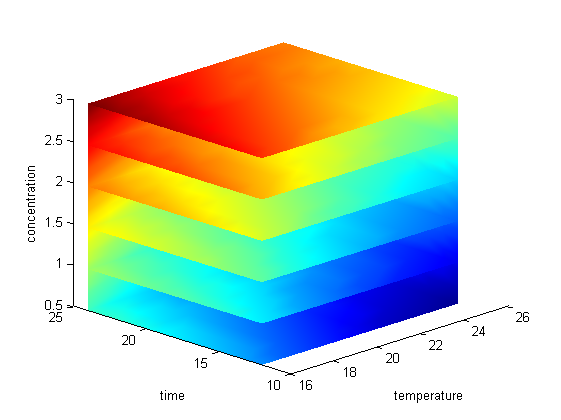Figure 5: 4-D slice picture of Relative Enzyme Activity

2.4 Conclusion
We established the multivariate nonlinear regression analysis model established based on experimental data and find the mathematical expression of the efficiency of recombination cell decomposing PET. The optimum condition we find of this group is: x1=25h, x2=16℃, x3=3ml. So we can do more experiment near this condition in the future.
Reference
 Barth, Markus; Oeser, Thorsten; Wei, Ren. Effect of hydrolysis products on the enzymatic degradation of polyethylene terephthalate nanoparticles by a polyester hydrolase from Thermobifida fusca. BIOCHEMICAL ENGINEERING JOURNAL, 93, 222-228, JAN 15 2015.
 Markus Barth, Ren Wei, Thorsten Oeser, Johannes Then, Juliane Schmidt, Franziska Wohlgemuth, Wolfgang Zimmermann. Enzymatic hydrolysis of polyethylene terephthalate films in an ultrafiltration membrane reactor. Journal of Membrane Science 494 (2015) 182–187.
 Ren Wei a, Thorsten Oeser a, Markus Barth a, Nancy Weigl a, Anja Lübs b, Michaela Schulz-Siegmund b, Michael C. Hacker b, Wolfgang Zimmermann. Turbidimetric analysis of the enzymatic hydrolysis of polyethylene terephthalate nanoparticles. Journal of Molecular Catalysis B: Enzymatic 103 (2014) 72–78.
 Ren Wei, Thorsten Oeser, Juliane Schmidt, Ren e Meier, Markus Barth, Johannes Then, Wolfgang Zimmermann. Engineered Bacterial Polyester Hydrolases Efficiently Degrade Polyethylene Terephthalate. Biotechnology and Bioengineering, Vol. 9999, No. xxx, 2016.
 Pan Wang & Jie He & Yufei Sun & Matthew Reynolds & Li Zhang & Shuangyan Han & Shuli Liang1 & Haixin Sui & Ying Lin. Display of fungal hydrophobin on the Pichia pastoris cell surface and its influence on Candida antarctica lipase B Due to Relieved Product Inhibition. Appl Microbiol Biotechnol, 12 March 2016.
 Shosuke Yoshida, Kazumi Hiraga, Toshihiko Takehana, Ikuo Taniguchi, Hironao Yamaji, Yasuhito Maeda, Kiyotsuna Toyohara, Kenji Miyamoto, Yoshiharu Kimura, Kohei Oda. A bacterium that degrades and assimilates poly (ethylene terephthalate). BIODEGRADATION 11 MARCH 2016• VOL 351 ISSUE 6278.